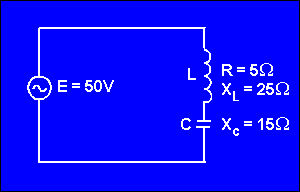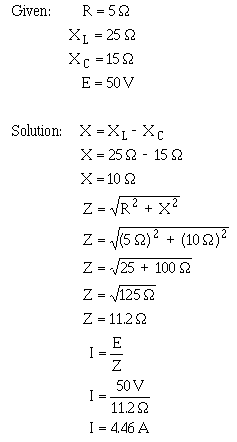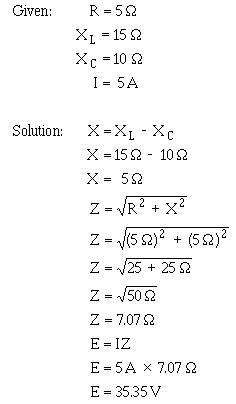Ohms law for AC impedance, Z, represents the combined opposition of all the reactances and resistances, this general law for ac is">Custom SearchOHMS LAW FOR AC In general, Ohm's law cannot be applied to alternating-current circuits since it does not consider the reactance which is always present in such circuits. However, by a modification of Ohm's law which does take into consideration the effect of reactance we obtain a general law which is applicable to ac circuits. Because the impedance, Z, represents the combined opposition of all the reactances and resistances, this general law for ac is,This general modification applies to alternating current flowing in any circuit, and any one of the values may be found from the equation if the others are known. For example, suppose a series circuit contains an inductor having 5 ohms resistance and 25 ohms inductive reactance in series with a capacitor having 15 ohms capacitive reactance. If the voltage is 50 volts, what is the current? This circuit can be drawn as shown in figure 4-8. Figure 4-8. - Series LC circuit.Now suppose the circuit contains an inductor having 5 ohms resistance and 15 ohms inductive reactance in series with a capacitor having 10 ohms capacitive reactance. If the current is 5 amperes, what is the voltage?Q.18 What are the Ohm's law formulas used in an ac circuit to determine voltage and current?Integrated Publishing, Inc. - A (SDVOSB) Service Disabled Veteran Owned Small Business